# sklearn.datasets.load_digits¶

Load and return the digits dataset (classification).

Each datapoint is a 8x8 image of a digit.

 Classes 10 Samples per class ~180 Samples total 1797 Dimensionality 64 Features integers 0-16

This is a copy of the test set of the UCI ML hand-written digits datasets https://archive.ics.uci.edu/ml/datasets/Optical+Recognition+of+Handwritten+Digits

Read more in the User Guide.

Parameters:
n_classint, default=10

The number of classes to return. Between 0 and 10.

return_X_ybool, default=False

If True, returns (data, target) instead of a Bunch object. See below for more information about the data and target object.

New in version 0.18.

as_framebool, default=False

If True, the data is a pandas DataFrame including columns with appropriate dtypes (numeric). The target is a pandas DataFrame or Series depending on the number of target columns. If return_X_y is True, then (data, target) will be pandas DataFrames or Series as described below.

New in version 0.23.

Returns:
dataBunch

Dictionary-like object, with the following attributes.

data{ndarray, dataframe} of shape (1797, 64)

The flattened data matrix. If as_frame=True, data will be a pandas DataFrame.

target: {ndarray, Series} of shape (1797,)

The classification target. If as_frame=True, target will be a pandas Series.

feature_names: list

The names of the dataset columns.

target_names: list

The names of target classes.

New in version 0.20.

frame: DataFrame of shape (1797, 65)

Only present when as_frame=True. DataFrame with data and target.

New in version 0.23.

images: {ndarray} of shape (1797, 8, 8)

The raw image data.

DESCR: str

The full description of the dataset.

(data, target)tuple if return_X_y is True

A tuple of two ndarrays by default. The first contains a 2D ndarray of shape (1797, 64) with each row representing one sample and each column representing the features. The second ndarray of shape (1797) contains the target samples. If as_frame=True, both arrays are pandas objects, i.e. X a dataframe and y a series.

New in version 0.18.

Examples

To load the data and visualize the images:

>>> from sklearn.datasets import load_digits
>>> print(digits.data.shape)
(1797, 64)
>>> import matplotlib.pyplot as plt
>>> plt.gray()
>>> plt.matshow(digits.images)
<...>
>>> plt.show()


## Examples using sklearn.datasets.load_digits¶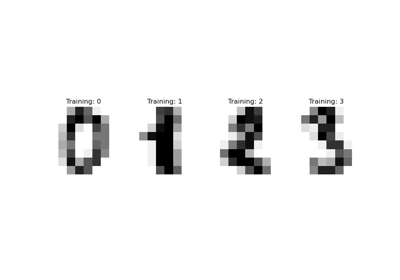Recognizing hand-written digits

Recognizing hand-written digits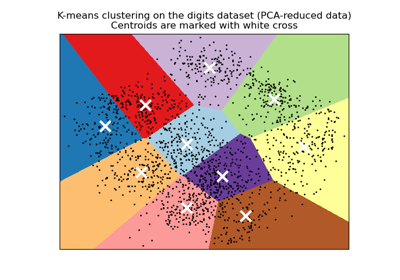A demo of K-Means clustering on the handwritten digits data

A demo of K-Means clustering on the handwritten digits data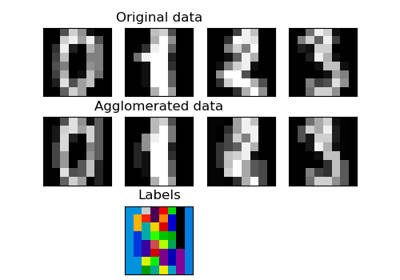Feature agglomeration

Feature agglomeration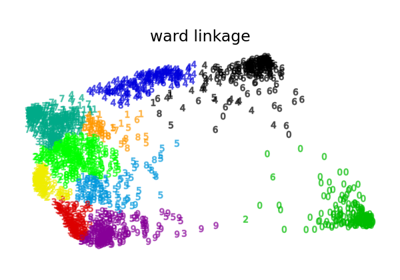Various Agglomerative Clustering on a 2D embedding of digits

Various Agglomerative Clustering on a 2D embedding of digits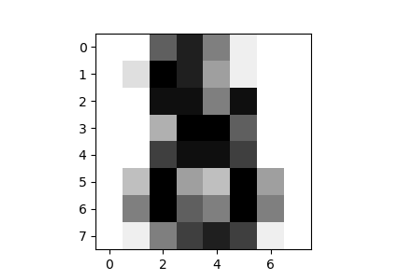The Digit Dataset

The Digit Dataset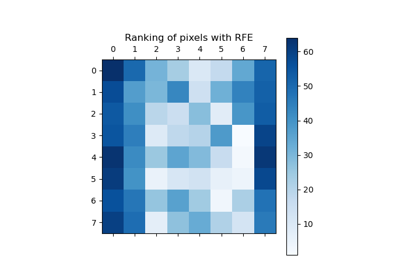Recursive feature elimination

Recursive feature elimination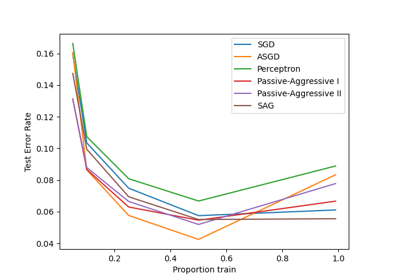Comparing various online solvers

Comparing various online solvers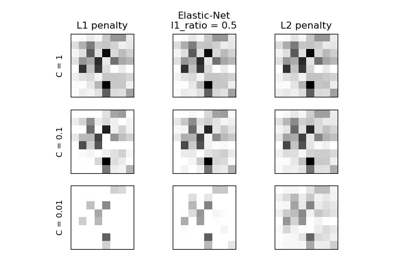L1 Penalty and Sparsity in Logistic Regression

L1 Penalty and Sparsity in Logistic Regression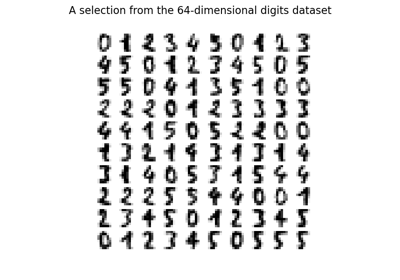Manifold learning on handwritten digits: Locally Linear Embedding, Isomap…

Manifold learning on handwritten digits: Locally Linear Embedding, Isomap...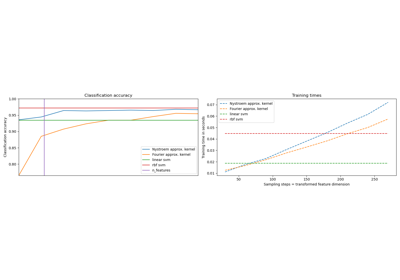Explicit feature map approximation for RBF kernels

Explicit feature map approximation for RBF kernels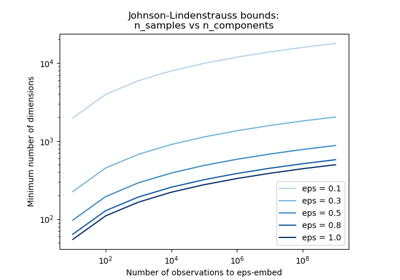The Johnson-Lindenstrauss bound for embedding with random projections

The Johnson-Lindenstrauss bound for embedding with random projections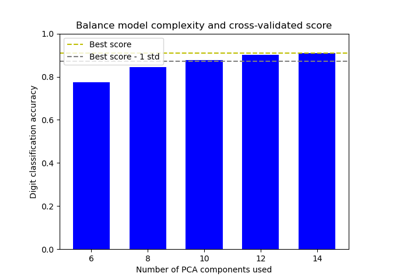Balance model complexity and cross-validated score

Balance model complexity and cross-validated scoreComparing randomized search and grid search for hyperparameter estimation

Comparing randomized search and grid search for hyperparameter estimationCustom refit strategy of a grid search with cross-validation

Custom refit strategy of a grid search with cross-validation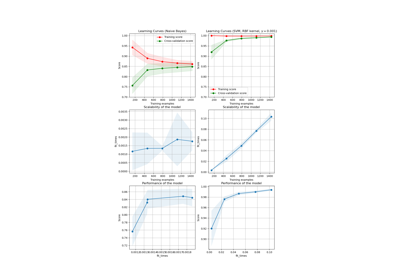Plotting Learning Curves

Plotting Learning Curves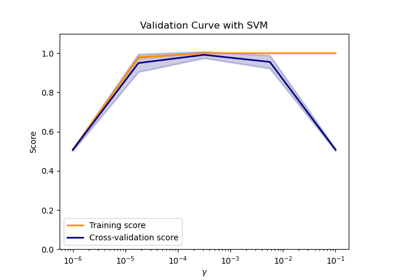Plotting Validation Curves

Plotting Validation Curves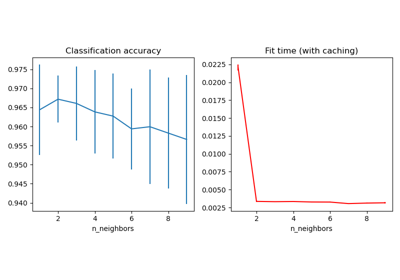Caching nearest neighbors

Caching nearest neighbors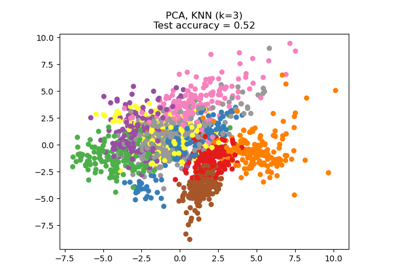Dimensionality Reduction with Neighborhood Components Analysis

Dimensionality Reduction with Neighborhood Components Analysis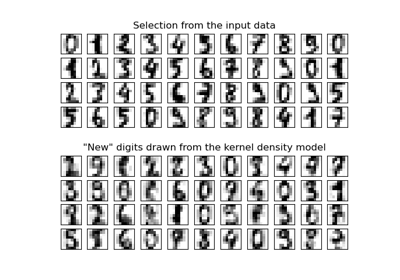Kernel Density Estimation

Kernel Density Estimation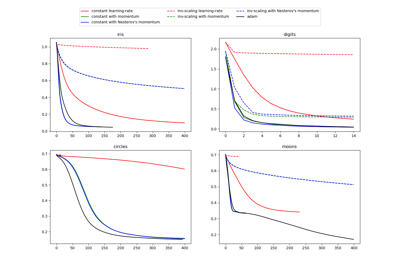Compare Stochastic learning strategies for MLPClassifier

Compare Stochastic learning strategies for MLPClassifier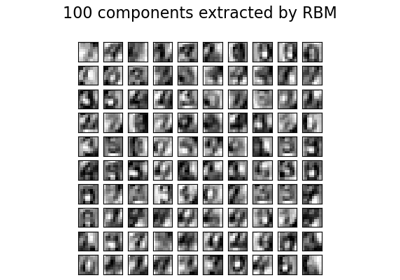Restricted Boltzmann Machine features for digit classification

Restricted Boltzmann Machine features for digit classification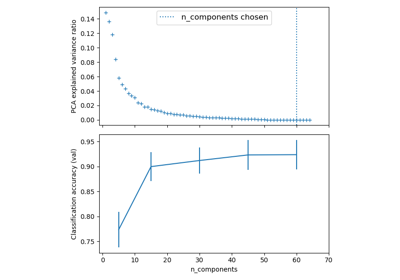Pipelining: chaining a PCA and a logistic regression

Pipelining: chaining a PCA and a logistic regression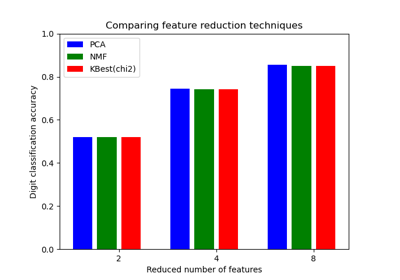Selecting dimensionality reduction with Pipeline and GridSearchCV

Selecting dimensionality reduction with Pipeline and GridSearchCV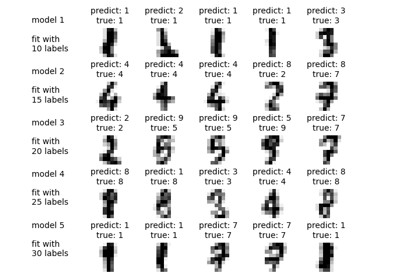Label Propagation digits active learning

Label Propagation digits active learning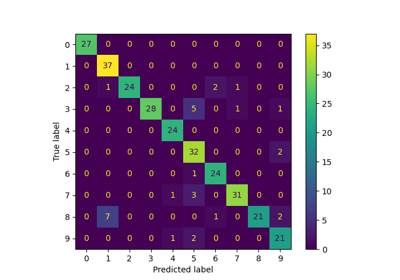Label Propagation digits: Demonstrating performance

Label Propagation digits: Demonstrating performance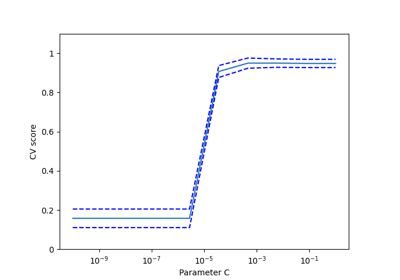Cross-validation on Digits Dataset Exercise

Cross-validation on Digits Dataset ExerciseDigits Classification Exercise

Digits Classification Exercise